DECIMALS (Exercise – 8.1)EX 8.1 QUESTION 1.

Write the following as numbers in the given table.Solution:

 Rows Hundreds Tens Ones Tenths a 0 3 1 2 b 1 1 0 4

EX 8.1 QUESTION 2.

Write the following decimals in the place value table.

(a) 19.4

(b) 0.3

(c) 10.6

(d) 205.9

Solutions:

 Hundreds Tens Ones Tenths 19.4 0 1 9 4 0.3 0 0 0 3 10.6 0 1 0 6 205.9 2 0 5 9

EX 8.1 QUESTION 3.

Write each of the following as decimals:

(a) Seven-tenths

(b) Two tens and nine-tenths

(c) Fourteen point six

(d) One hundred and two ones

(e) Six hundred point eight

Solutions:

(a) Seven-tenths = 7 / 10 = 0.7

(b) Two tens and nine-tenths = 20 + 9 / 10 = 20.9

(c) Fourteen point six = 14.6

(d) One hundred and two ones =100 + 2 = 102.0

(e) Six hundred point eight = 600.8

EX 8.1 QUESTION 4.

Write each of the following as decimals:

(a) 5 / 10

(b) 3 + 7 / 10

(c) 200 + 60 + 5 + 1 / 10

(d) 70 + 8 / 10

(e) 88 / 10

(f)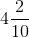(g) 3 / 2

(h) 2 / 5

(i) 12 / 5

(j)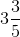(k)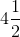Solutions:

(a) 5 / 10 = 0.5

(b) 3 + 7 / 10 = 3 + 0.7

= 3.7

(c) 200 + 60 + 5 + 1 / 10 = 265 + 0.1

= 265.1

(d) 70 + 8 / 10 = 70 + 0.8

= 70.8

(e) 88 / 10 = 80 / 10 + 8 / 10

= 8 + 0.8

= 8.8

(f)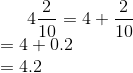(g) 3 / 2 = (2 + 1) / 2

= 2 / 2 + 1 / 2

= 1 + 0.5

= 1.5

(h) 2 / 5 = 0.4

(i) 12 / 5 = (10 + 2) / 5

= 10 / 5 + 2 / 5

= 2 + 0.4

= 2.4

(j)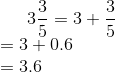(k)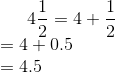EX 8.1 QUESTION 5.

Write the following decimals as fractions. Reduce the fraction to lowest form.

(a) 0.6

(b) 2.5

(c) 1.0

(d) 3.8

(e) 13.7

(f) 21.2

(g) 6.4

Solutions:

(a) 0.6 = 6 / 10

= 3 / 5

(b) 2.5 = 25 / 10

= 5 / 2

(c) 1.0 = 1

= 1

(d) 3.8 = 38 / 10

= 19 / 5

(e) 13. 7 = 137 / 10

(f) 21.2 = 212 / 10

= 106 / 5

(g) 6.4 = 64 / 10

= 32 / 5

EX 8.1 QUESTION 6.

Express the following as cm using decimals.

(a) 2 mm

(b) 30 mm

(c) 116 mm

(d) 4 cm 2 mm

(e) 162 mm

(f) 83 mm

Solutions:

We know that

1 cm = 10 mm

1 mm = 1 / 10 cm

(a) 2 mm = 2 / 10 cm

= 0.2 cm

(b) 30 mm = 30 / 10 cm

= 3.0 cm

(c) 116 mm = 116 / 10 cm

= 11.6 cm

(d) 4 cm 2 mm = [(4 + 2 / 10)] cm

= 4.2 cm

(e) 162 mm = 162 / 10 cm

= 16.2 cm

(f) 83 mm = 83 / 10 cm

= 8.3 cm

EX 8.1 QUESTION 7.

Between which two whole numbers on the number line are the given numbers lie? Which of these whole numbers is nearer the number?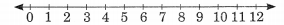(a) 0.8
(b) 5.1
(c) 2.6
(d) 6.4
(e) 9.1
(f) 4.9
Solution:
(a) 0.8 lies between  0 and 1.
(a) 5.1 lies between  5 and 6.
(b) 2.6 lies between  2 and 3.
(c) 6.4 lies between  6 and 7.
(d) 9.1 lies between  9 and 10.
(e) 4.9 lies between  4 and 5.

EX 8.1 QUESTION 8.

Show the following numbers on the number line.
(a) 0.2
(b) 1.9
(c) 1.1
(d) 2.5
Solution:EX 8.1 QUESTION 9.

Write the decimal number represented by the points A, B, C, D on the given number line.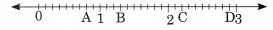Solution:
Point A represents 0.8
Point B represents 1.3
Point C represents 2.2
Point D represents 2.9

EX 8.1 QUESTION 10.

(a) The length of Ramesh’s notebook is 9 cm 5mm. What will be its length in cm?
(b) The length of a young gram plant is 65 mm. Express its length in cm.
Solution:

The length of Ramesh notebook is 9 cm 5 mm

The length in cm is [(9 + 5 / 10)] cm

= 9.5 cm

(b) The length of a gram plant is 65 mm

Hence, the length in cm is 65 / 10

= 6.5 cm